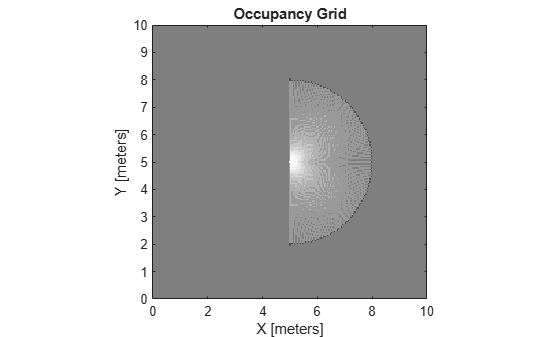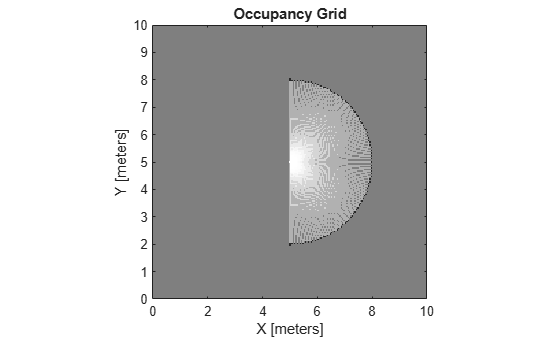# getOccupancy

Get occupancy value of locations

## Syntax

``occVal = getOccupancy(map,xy)``
``occVal = getOccupancy(map,xy,'local')``
``occVal = getOccupancy(map,ij,'grid')``
``[occVal,validPts] = getOccupancy(___)``
``occMatrix = getOccupancy(map)``
``occMatrix = getOccupancy(map,bottomLeft,matSize)``
``occMatrix = getOccupancy(map,bottomLeft,matSize,'local')``
``occMatrix = getOccupancy(map,topLeft,matSize,'grid')``

## Description

example

````occVal = getOccupancy(map,xy)` returns an array of probability occupancy values at the `xy` locations in the world frame. Values close to 1 represent a high probability that the cell contains an obstacle. Values close to 0 represent a high probability that the cell is not occupied and obstacle free. Unknown locations, including outside the map, return `map.DefaultValue`.```
````occVal = getOccupancy(map,xy,'local')` returns an array of occupancy values at the `xy` locations in the local frame.```
````occVal = getOccupancy(map,ij,'grid')` specifies `ij` grid cell indices instead of `xy` locations.```
````[occVal,validPts] = getOccupancy(___)` additionally outputs an `n`-element vector of logical values indicating whether input coordinates are within the map limits.```
````occMatrix = getOccupancy(map)` returns all occupancy values in the map as a matrix.```
````occMatrix = getOccupancy(map,bottomLeft,matSize)` returns a matrix of occupancy values by specifying the bottom-left corner location in world coordinates and the matrix size in meters.```
````occMatrix = getOccupancy(map,bottomLeft,matSize,'local')` returns a matrix of occupancy values by specifying the bottom-left corner location in local coordinates and the matrix size in meters.```
````occMatrix = getOccupancy(map,topLeft,matSize,'grid')` returns a matrix of occupancy values by specifying the top-left corner location in grid indices and the matrix size.```

## Examples

collapse all

Create an empty occupancy grid map.

`map = occupancyMap(10,10,20);`

Input pose of the vehicle, ranges, angles, and the maximum range of the laser scan.

```pose = [5,5,0]; ranges = 3*ones(100,1); angles = linspace(-pi/2,pi/2,100); maxrange = 20;```

Create a `lidarScan` object with the specified ranges and angles.

`scan = lidarScan(ranges,angles);`

Insert the laser scan data into the occupancy map.

`insertRay(map,pose,scan,maxrange);`

Show the map to see the results of inserting the laser scan.

`show(map)`Check the occupancy of the spot directly in front of the vehicle.

`getOccupancy(map,[8 5])`
```ans = 0.7000 ```

Add a second reading and view the update to the occupancy values. The additional reading increases the confidence in the readings. The free and occupied values become more distinct.

```insertRay(map,pose,scan,maxrange); show(map)````getOccupancy(map,[8 5])`
```ans = 0.8448 ```

Access occupancy values and check their occupancy status based on the occupied and free thresholds of the `occupancyMap` object.

Create a matrix and populate it with values. Use this matrix to create an occupancy map.

```p = 0.5*ones(20,20); p(11:20,11:20) = 0.75*ones(10,10); map = occupancyMap(p,10);```

Get the occupancy of different locations and check their occupancy statuses. The occupancy status returns 0 for free space and 1 for occupied space. Unknown values return –1.

`pocc = getOccupancy(map,[1.5 1])`
```pocc = 0.7500 ```
`occupied = checkOccupancy(map,[1.5 1])`
```occupied = 1 ```
`pocc2 = getOccupancy(map,[5 5],'grid')`
```pocc2 = 0.5000 ```
`occupied2 = checkOccupancy(map,[5 5],'grid')`
```occupied2 = -1 ```

## Input Arguments

collapse all

Map representation, specified as a `occupancyMap` object. This object represents the environment of the vehicle. The object contains a matrix grid with values representing the probability of the occupancy of that cell. Values close to 1 represent a high probability that the cell contains an obstacle. Values close to 0 represent a high probability that the cell is not occupied and obstacle free.

World coordinates, specified as an n-by-2 matrix of ```[x y]``` pairs, where n is the number of world coordinates.

Data Types: `double`

Grid positions, specified as an n-by-2 matrix of ```[i j]``` pairs in ```[rows cols]``` format, where n is the number of grid positions.

Data Types: `double`

Location of bottom left corner of output matrix in world or local coordinates, specified as a two-element vector, ```[xCoord yCoord]```. Location is in world or local coordinates based on syntax.

Data Types: `double`

Output matrix size, specified as a two-element vector, ```[xLength yLength]``` or `[gridRow gridCol]`. Size is in world, local, or grid coordinates based on syntax.

Data Types: `double`

Location of top left corner of grid, specified as a two-element vector, `[iCoord jCoord]`.

Data Types: `double`

## Output Arguments

collapse all

Probability occupancy values, returned as a column vector the same length as either `xy` or `ij`.

Values close to 0 represent a high probability that the cell is not occupied and obstacle free.

Valid map locations, returned as an n-by-1 column vector equal in length to `xy` or `ij`. Locations inside the map return a value of 1. Locations outside the map limits return a value of 0.

Matrix of occupancy values, returned as matrix with size equal to `matSize` or the size of `map`.

Values close to 0 represent a high probability that the cell is not occupied and obstacle free.

## Limitations

Occupancy values have a limited resolution of ±0.001. The values are stored as `int16` using a log-odds representation. This data type limits resolution, but saves memory when storing large maps in MATLAB®. When calling `setOccupancy` and then `getOccupancy`, the value returned might not equal the value you set. For more information, see the log-odds representations section in Occupancy Grids.

## Version History

Introduced in R2019b